## Example Questions

← Previous 1

### Example Question #1 : How To Find Mode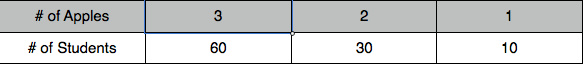Looking at the table, what is the difference of the mean minus the mode.

–0.5

1.0

0.5

–1.0

–0.5

Explanation:

The mode is 3 and subtracting 3 from 2.5 gives -0.5.

### Example Question #2 : How To Find Mode

Find the mode of the following set of numbers:

1, 6, 3, 6, 5, 9

8

6

9

5

6

Explanation:

Mode is the most frequently occuring number in a set. 6 appears most frequently since there are 2 sixes but the rest of the numbers only appear once. The mode is 6

### Example Question #71 : Statistics

Find the mode in this set of numbers:

2, 100, 52, 97, 1, 7, 22, 19, 100

100

1

2

none of these

97

100

Explanation:

The mode is the number that appears the most

### Example Question #4 : How To Find Mode

Find the mode from the following set of numbers

1, 4, 8, 17, 8, 8, 15, 21, 32, 17

21

8

17

4

15

8

Explanation:

The mode is the number that appears the most in a given set of numbers

### Example Question #5 : How To Find Mode

Find the mode.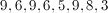Explanation:

The mode is the number that appears the most often. 9 appears three times.

### Example Question #6 : How To Find Mode

What is the mode of the following set of numbers?

24, 38, 26, 27, 7, 27, 34, 12, 13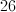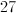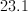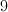Explanation:

The mode is the number that appears the most in a given set of numbers.

### Example Question #101 : Statistics

Consider the following dataset: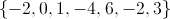The arithmetic mean of the dataset is.

The median of the dataset is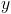.

The range of the dataset is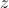.

The mode of the dataset is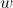.

Which of the following statements is true?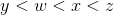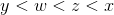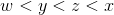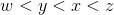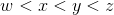Explanation:

In general: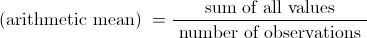In this case the mean is: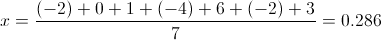To obtain the median, arrange the observations from smallest to largest: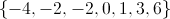When there is an odd number of observations, the median is the middle value. In this case: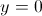The range is the difference between the largest and smallest value: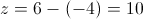The mode is the value that occurs most often in the dataset: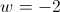Since: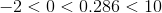we can conclude:### Example Question #1 : How To Find Mode

What is the mode of the data set?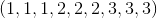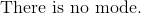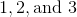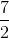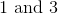Explanation:

The mode is the most frequent number or numbers that happen in a set of numbers.  There can be more than one mode.

The data set is already in order from smallest to largest.  Since there are three of each number, 1,2 and 3, all of the numbers are considered to be the mode.

The correct answer is: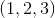### Example Question #1 : How To Find Mode

What is the mode in this set?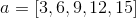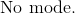Explanation:

The definition of mode is the numbers with the highest frequency in the set.

Since there is only one frequency of every number in this dataset, there will be no mode existent in the set.

The answer is:### Example Question #1 : Mode

Find the mode of the following set of numbers:

1,5,14,17,22,23,23